Aptitude Tests 4 Me

Non Verbal Logic

Detailed solution

101.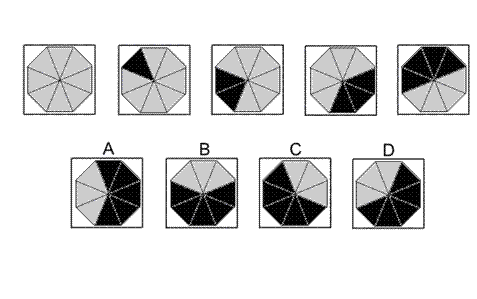Solution: D Explanation: In this question, from each diagram to the next diagram of the sequence, follow these rules: 1. Change the colour of the top triangle to black and rotate the octagon by 45° anticlockwise. 2. Change the colour of the adjacent triangle (in a clockwise sense) to the black one also to black and rotate the octagon by 90° anticlockwise. 3. Change the colour of the adjacent triangle (in a clockwise sense) to the two black ones also to black and rotate the octagon by 135° anticlockwise. etc Following this pattern, for the next diagram of the sequence we should change the colour of the adjacent triangle (in a clockwise sense) to the four black ones also to black and rotate the octagon by 225°. The correct answer, therefore, is D.

102.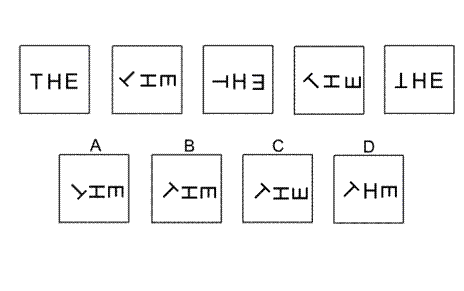Solution: B Explanation: In this question, each of the three letters - T, H and E follows its own rule. The T rotates anticlockwise by 135° each time. Following this rule, the correct answer could be B, C or D. The H rotates by 90° each time (clockwise or anticlockwise, it doesn't matter which). When this rule is also applied, the correct answer could be B or C. The E rotates by 90° clockwise each time. When this rule is also applied, the correct answer must be B.

103.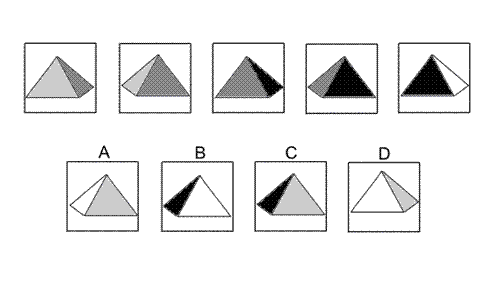Solution: B Explanation: In this question there is a square-based pyramid with its four triangular faces coloured light-grey, dark-grey, black and white. From one diagram to the next the pyramid rotates around its vertical axis by 45° each time. For the next diagram of the sequence the two faces that are seen will be the same as in the previous diagram - black and white - but rotated by 45°. The correct answer, therefore, is B.

104.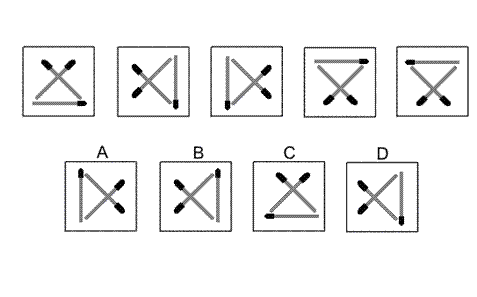Solution: A Explanation: In this question the three matchsticks reflect alternately in two axes. The first axis is along the line of the matchstick lying diagonally from the top left to the bottom right. The second axis is the vertical axis. For the next diagram of the sequence, therefore, we should reflect in the first axis and the answer is A.

105.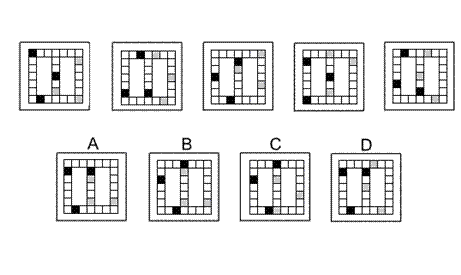Solution: C Explanation: In this question, the black squares move around the left-hand circuit, moving two places clockwise each time; and the grey squares move around the right-hand circuit, moving three places anticlockwise each time. Following these rules, the next diagram in the sequence must be C.

 1 2 3 4 5 6 7 8 9 10 11 12 13 14 15 16 17 18 19 20 21 22 23 24 25 26 27 28 29 30 31 32 33 34 35 36 37 38 39 40 41 42 43 44 45 46 47 48 49 50 51 52 53 54 55 56 57 58 59 60 61 62 63 64 65 66 67 68 69 70 71 72 73 74 75 76 77 78 79 80 81 82 83 84 85 86 87 88Passage ReadingVerbal LogicNon Verbal LogicNumerical LogicData InterpretationReasoningAnalytical AbilityBasic NumeracyAbout UsContactPrivacy PolicyMajor TestsFAQ